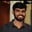Related Tags

c
communitycreator

# How to add two matrices in CBuchiredddypalli Koushik

### Overview

In this shot, we’ll see how we can add two matrices in the C programming language.

To do that, we need to read two matrices using the standard input (scanf) and add the two matrices to get the result. This result should be stored in the new matrix.

### Example

For a 2x2 matrix, we can give the values of 2 matrices as the following: 2 1 2 3 4 5 6 7 8.

Here, 2 is the value of n—the first four values for the first matrix and the next four values for the second matrix.

Matrix 1:

$\begin{bmatrix} 1 & 2 \\ 3 & 4\\ \end{bmatrix} \quad$

Matrix 2:

$\begin{bmatrix} 5 & 6 \\ 7 & 8\\ \end{bmatrix} \quad$

Result:

$\begin{bmatrix} 6 & 8 \\ 10 & 12\\ \end{bmatrix} \quad$

Note: The first point we have to keep in mind is that we can add both square matrices and rectangular matrices. The addition of two matrices is possible only when they are of the same order. In other words, both the matrices should be of the same size.

### Algorithm

The addition of two matrices means that we need to add the corresponding elements of the two matrices.

When reading the data, we need to use loops.

We also need loops to access the corresponding elements of the matrices. After accessing the elements, we’ll add the elements and store the data in the resultant matrix.

The pseudocode looks like this:

for(i=0; i<n ;i++)
{
for(j=0; j<n ;j++)
{
Resultant[i][j] = Matrix1[i][j] + Matrix2[i][j];
}
}


The algorithm is explained for 2x2 dimension matrices in the slides below.

The original matrices initially
1 of 5

### Example

Note: To run the code below, first give the input value of n, which represents the size of the matrix. Then read the two matrices which you want to add.

For a 2x2 matrix, we can give the values of 2 matrices as the following: 2 1 2 3 4 5 6 7 8. Here, 2 is the value of n—the first four values for the first matrix and the next four values for the second matrix.

After giving all the inputs, we add the two matrices and store them in the resultant matrix.

main.c
file__ed_input.txt
#include<stdio.h>
int main()
{
int i,j,n;
scanf("%d",&n);
printf("The value of n is : %d\n",n);
int Matrix1[n][n],Matrix2[n][n],Resultant[n][n];
for(i=0;i<n;i++)
{
for(j=0;j<n;j++)
{
scanf("%d",&Matrix1[i][j]);
}
}

for(i=0;i<n;i++)
{
for(j=0;j<n;j++)
{
scanf("%d",&Matrix2[i][j]);
}
}

for(i=0;i<n;i++)
{
for(j=0;j<n;j++)
{
Resultant[i][j]=Matrix1[i][j]+Matrix2[i][j];

}
}
printf("The Resultant Matrix is :\n");
for(i=0;i<n;i++)
{
for(j=0;j<n;j++)
{

printf("%d ",Resultant[i][j]);
}
printf("\n");
}
}

Enter the input below

### Explanation

• The n represents the dimensions of the matrix.
• The Matrix1[n][n] represents the Matrix 1.
• The Matrix2[n][n] represent the Matrix 2.
• The Resultant[n][n] represents the addition of two matrices or the resultant matrix.
• Line 4: We take the value of n, which represents the size of the matrix.

• Lines 8–22: We read the first matrix and the second matrix using the for loop.

• Lines 24-31: We use a for loop and add both the matrices. This addition of two matrices is put into the resultant matrix.

• Lines 32–42: We print the final matrix.

RELATED TAGS

c
communitycreator

CONTRIBUTORBuchiredddypalli Koushik
RELATED COURSES

View all Courses

Keep Exploring

Learn in-demand tech skills in half the time